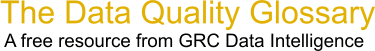Choose Index below for a list of all words and phrases defined in this glossary.

# Compass Rule

index | Index

## Compass Rule - definition(s)

compass rule - [surveying] A widely used rule for adjusting a traverse that assumes the precision in angles or directions is equivalent to the precision in distances. This rule distributes the closure error over the whole traverse by changing the northings and eastings of each traverse point in proportion to the distance from the beginning of the traverse. More specifically, a correction factor is computed for each point as the sum of the distances along the traverse from the first point to the point in question, divided by the total length of the traverse. The correction factor at each point is multiplied by the overall closure error to get the amount of error correction distributed to the point's coordinates. The compass rule is also known as the Bowditch rule, named for the American mathematician and navigator Nathaniel Bowditch (1773-1838).

[Category=Geospatial ]

Source: esri, 26 February 2012 09:14:36, http://support.esri.com/en/knowledgebase/GISDictionary/term/abbreviationData Quality Glossary.  A free resource from GRC Data Intelligence. For comments, questions or feedback: dqglossary@grcdi.nl